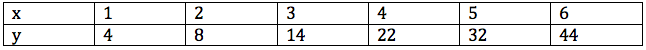Question 128

#In the above table, for suitably chosen constants a, b and c, which one of the following best describes the relation between y and x?

Solution

y=a+bx is a linear function and clearly the function shown in the table is not linear.
Lets take option B:

Suppose $$y=f(x)=a+bx+cx^2$$
f(1)=4 => a+b+c=4 -- (1)
f(2)=8 => a+2b+4c=8 -- (2)
f(3)=14 => a+3b+9c=14 -- (3)

Let us solve the equations and see if $$f(4), f(5),f(6)$$ also satisfy the given equation.

(2)-(1) => b+3c=4
(3)-(2) => b+5c=6
This gives c=1 and b=1 ==> a=2

So, $$f(x)=2+x+x^2$$
$$f(4)=2+4+4^2 = 22$$
$$f(5)=2+5+5^2 = 32$$
$$f(6)=2+6+6^2 = 44$$

As all the three also satisfy the numbers given in the table, it can be inferred that the relationship between x and y is quadratic and the correct option is option (b)